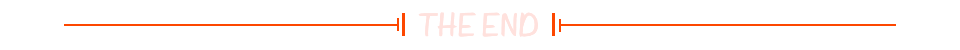# 【Vue】科学计数法常见处理

## 一、Vue之Number

在Vue中，可以使用JavaScript的内置Number对象来进行数字相关的操作和转换。

### 1、常见的Number对象的用法示例

``````<template>
<div>
<p>{{ formattedNumber }}</p>
</div>
</template>

<script>
export default {
data() {
return {
number: 123456.789,
};
},
computed: {
formattedNumber() {
const formatted = Number(this.number).toLocaleString(); // 格式化为带千分位的字符串
const rounded = Number(this.number).toFixed(2); // 保留两位小数
const parsed = Number.parseFloat("3.14"); // 解析字符串为浮点数
const intValue = Number.parseInt("42"); // 解析字符串为整数
const isFiniteNumber = Number.isFinite(123); // 检查是否为有限数
const isNaNNumber = Number.isNaN(NaN); // 检查是否为NaN
return `\${formatted}, \${rounded}, \${parsed}, \${intValue}, \${isFiniteNumber}, \${isNaNNumber}`;
},
},
};
</script>
``````

### 2、常用方法和属性总结

• toLocaleString()：将数字格式化为带有千分位分隔符的字符串。
• toFixed()：将数字保留指定的小数位数，并返回一个字符串。
• parseFloat()：将字符串解析为浮点数。
• parseInt()：将字符串解析为整数。
• isFinite()：检查一个值是否为有限数。
• isNaN()：检查一个值是否为NaN。

在计算属性formattedNumber中，我们将这些方法应用到number数据上，并返回一个包含了格式化、保留小数、解析字符串、检查数值的结果的字符串。

请注意，这只是一些Number对象的用法示例，你可以根据自己的需求进行修改和扩展。

## 二、Vue 科学计数法互相转换

在Vue中，可以使用JavaScript的内置方法toExponential()来处理科学计数法，并结合toFixed()方法来保留指定小数位数。

以下是一个示例代码，将科学计数法转换为对应保留两位小数的字符串：

``````<template>
<div>
<p>{{ formattedNumber }}</p>
</div>
</template>

<script>
export default {
data() {
return {
number: 1.23e+6,
};
},
computed: {
formattedNumber() {
const decimalNumber = this.number.toFixed(2); // 保留两位小数
const scientificNotation = Number(decimalNumber).toExponential(); // 转换为科学计数法
return scientificNotation;
},
},
};
</script>``````

在上述示例中，number的初始值为科学计数法表示的数字1.23e+6。通过toFixed(2)方法，我们将其转换为具有两位小数的字符串”1230000.00″。然后，使用Number()将其转换回数字，并使用toExponential()方法将其转换为科学计数法表示的字符串”1.23e+6″。

在模板中，我们通过formattedNumber计算属性将格式化后的科学计数法字符串显示出来。

请注意，这只是一个示例，你可以根据自己的需求进行修改和调整

## 三、举例

### 1、保留两位小数

``````    dealNum(val) {
return (val * 1).toFixed(2);
},``````

### 2、保留两位小数的百分数

``````    dealNum(val) {
return (val * 100).toFixed(2) + "%";
},``````

### 3、查询通过率

``````//通过率
dealNum(passNum,  totalNum) {
return (passNum / totalNum).toFixed(2) + "%";
},``````

## 四、往期相关优质推荐

 VSCode 最全实用插件（VIP典藏版） Vue超详细整理（VIP典藏版） Vue中created，mounted，updated详解 一文快速上手Echarts（持续更新） Vue中el-table数据项扩展各种类型总结（持续更新）(0)